Latest Banking jobs   »

# Reasoning Quiz For RBI Grade B Phase 1 2023-19th March

Direction (1-5): Study the following information carefully and answer the questions given below-
Eight persons P, Q, R, S, T, U, V, W go for a vacation in different months of a year viz. March, April, May, June, July, August, September, and October. S goes in a month which has 31 days. Three persons go for vacation in between R and S. P goes for a vacation immediately before T but not in a month which has 31 days. Only two persons go for vacation in between W and Q. T goes in a month before S. Only one person goes in between U and V. W goes before S. U does not go after Q.

Q1. Who among the following go for vacation in May?
(a) P
(b) S
(c) R
(d) T
(e) None of these

Q2. R goes for a vacation in which of the following month?
(a) August
(b) March
(c) June
(d) September
(e) None of these

Q3. How many persons go for vacation in between W and V?
(a) One
(b) Two
(c) Three
(d) Four
(e) None of the above

Q4. Four of the following are alike in a certain way so form a group, who among the following does not belong to that group?
(a) V
(b) S
(c) R
(d) T
(e) Q

Q5. Who among the following go for vacation in June?
(a) P
(b) U
(c) W
(d) T
(e) None of these

Directions (6-10): In each of the questions below are given Some statements followed by Some Conclusions. You have to take the given statements to be true even if they seem to be at variance from commonly known facts. Read all the statements and then decide which of the given Conclusions logically follows from the given statements disregarding commonly known facts.

Q6.Statements: All Chocolates are Toffee.
Some Toffee are Lollipop.
Some Candy are Lollipop.

Conclusions: I. Some Toffee are Chocolates.
II. All Lollipop are Chocolates is a possibility.
III. Some Lollipop are not toffee.
(a) Only I follows
(b) Only II follows
(c) Only I and II follow
(d) Only III follows
(e) None follows

Q7. Statements: Some News are Impact.
Some Impact are Reactions.
All Reactions are Important.
Conclusions: I. Some Important are News is a possibility.
II. Some Important can be Impact.
III. All Reactions are not Impact.
(a) Only II follows
(b) Only II and III follow
(c) Only I and III follow
(d) Only I and II follow
(e) None of these

Q8. Statements: All Great are Achievement.
All Achievement are Stable.
All Focus are Stable.
Conclusions: I. Some Achievements can be Focus.
II. Some Stable are not Great.
III. All Great are Stable.
(a) Only I follows
(b) Only II follows
(c) Only III follows
(d) Only I and III follow
(e) None of these

Q9. Statements: Some Nice are Good.
All Good are Fine.
All Fine are Excellent.
Conclusions: I. Some Nice are Excellent.
II. Some Fine are not Good.
III. No Fine are Nice is a possibility.
(a) Only I follows
(b) Only II follows
(c) Only III follows
(d) Only I and II follow
(e) None of these

Q10. Statements: Some Straight are Line.
Some Straight are Diagonal.
Some Divide are Line.
Conclusions: I. Some Straight can be Divide.
II. Some Straight are not Diagonal.
III. Some Straight are Divide.
(a) Only I and III follow
(b) Only I follows
(c) Either I or III follow
(d) Only III follows
(e) None of these

Q11. If odd placed digits are decreased by 1 & even placed digits are increased by 1 in the number “1357986420”, then find the difference between sum of odd and even placed digits after rearrangement?
(a) 7
(b) 8
(c) 9
(d) 10
(e) None of the above

Q12. If all the digits of the number “012345678” are increased by 1, then find sum of all even digits after rearrangement?
(a) 20
(b) 24
(c) 22
(d) 18
(e) None of the above

Q13. If all the digits of the number “987654456789” are decreased by 1, then find sum of all odd digits after rearrangement?
a) 20
b) 30
c) 40
d) 50
e) None of the above

Q14. If all the digits of the number “014725836” are increased by 1, then find (sum of even placed digit value) / (sum of odd placed digit value) after rearrangement?
(a) 1/5
(b) 2/5
(c) 3/5
(d) 4/5
(e) None of the above

Q15. How many pairs of letters are there in the word “DIAGNOSTIC” which has as many letters between them as in the alphabetical series (both forward and backward direction)?
(a) None
(b) One
(c) Two
(d) Three
(e) Four

Solutions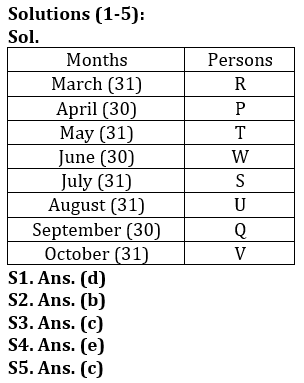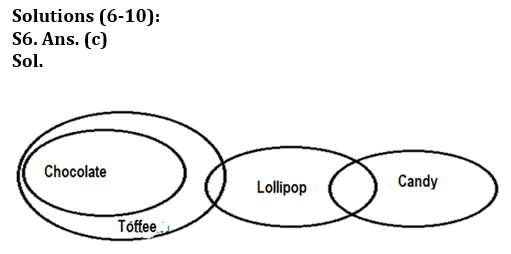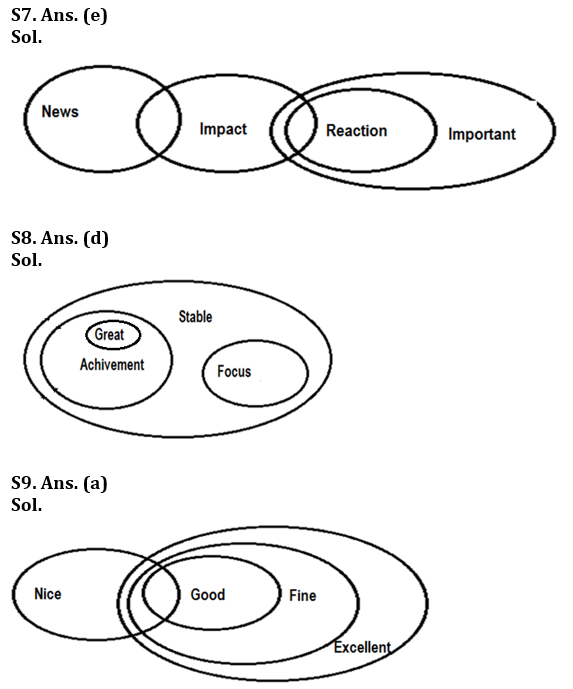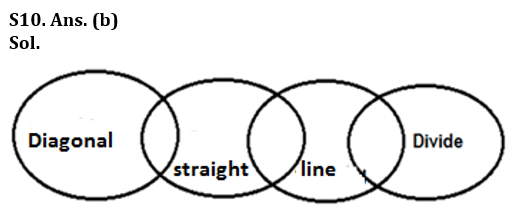S11. Ans. (c)
Sol. The number is 1357986420. After rearrangement, the number become 0448895511.
Sum of all odd placed digits = 0 + 4 + 8 + 5 + 1 = 18
Sum of all even placed digits = 4 + 8 + 9 + 5 + 1 = 27
So, difference between odd & even placed digits = 27 – 18 = 9

S12. Ans. (a)
Sol. The number is 012345678. After rearrangement, the number becomes 123456789.
Sum of all even digits = 2 + 4 + 6 + 8 = 20

S13. Ans. (b)
Sol. The number is 987654456789. After rearrangement, the number becomes 876543345678.
Sum of all odd digits = 7 + 5 + 3 + 3 + 5 + 7 = 30

S14. Ans. (d)
Sol. The number is 014725836. After rearrangement, the number becomes 125836947.
Sum of odd placed digits = 1 + 5 + 3 + 9 + 7 = 25
Sum of even placed digits = 2 + 8 + 6 + 4 = 20
Required value = 20/25 = 4/5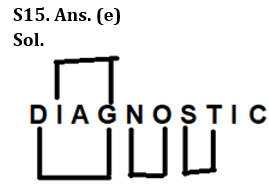## FAQs

### What is the selection process of the Bank Clerk?

There are 4 sections in the RBI Grade B Phase 1 Exam i.e. English Language, General Awareness, Quantitative Aptitude & Reasoning.

#### Congratulations!Union Budget 2023-24: Free PDF